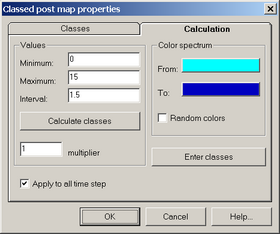﻿ Numerical modeling (ANSRADIAL) > Model editing > Graphic editor > Grouping

# Grouping

The "Classed post map properties" dialog box allows to find the maximal and minimal parameter value within the grid, group the values into classes (zones) and highlight them with color on the model.- call button in the graphic editor window."Classed post map properties" dialog boxes.

Explanatory table

 "Classes" tab Window for displaying and editing the outlined zones "№" button The number of the zone "Minimum" column Minimal value in the outlined zone (more than or equal to the prescribed value) "Maximum" column Maximal value in the outlined zone (less than the prescribed value) "Color" column Color of the outlined zone (double mouse click to change the color) "Number of classes" text box Enters the number of zones to be outlined: zones are both added and removed starting from the last one "Calculation" tab Prescribes the values and colors of outlined zones "Values" frame Prescribes values or calculate the outlined zones "Minimum" text box Minimal value for zones calculation "Maximum" text box Maximal value for zones calculation "Interval" text box Parameter intervals for zone outlining. For zone automatic calculation ("Calculate classes" button) the interval is specified based on minimal and maximal parameter values and specified number of zones "Calculate classes" button Automatically determines maximal and minimal values and calculates the interval "Multiplier" text box The factor by which each successive interval is multiplied to make zones irregular (=1 for regular /equal/ zones) "Color spectrum" frame Assign color to the outlined zones. Either gradation between two colors is used or random color is assigned to each zone "From" button Choose the first color for gradation "To" button Choose the last color for gradation "Random colors" option Option for assigning random color to each zone "Enter classes" button Displays the outlined zones ("Classes" tab) according to specified value intervals and assigned colors "Apply to all time steps" option Automatically calculates zones ("Calculate classes" button) and outline zones on the grid for all time steps. Is used only for output file